markov_decision_processes_and_dynamic_programming slides

Markov Decision Processes and Optimal Control¶

draft status

The agent is modeled to be in a state $s$ and can perform actions $a$.At each step $t$ the agent:

• Receives observation $o_t$
• Receives (immediate) scalar reward $R_t$
• Executes action $a_t$

The environment:

• Receives action $a_t$
• Emits observation $o_{t+1}$
• Emits scalar reward $R_{t+1}$

Here we assume that the environment is fully observable. So each observation corresponds to a state:

$$s_t = o_t$$

(If the environment is not fully observable we have a "Partially Observable Markov Decision Processes" POMDP.)

Markov Decision Process - Definition¶

A countable MDP (Markov Decision Process) is defined as a triple $\mathcal M = (\mathcal{S, A, P_0})$ with

• A countable not-empty set of states $s \in \mathcal S$.
• Countable not-empty set of actions $a \in \mathcal A$.
• Transition probability kernel $\mathcal P_0$: $\mathcal P_0$ assigns to each state-action pair $(S=s, A=a) \in \mathcal S \times \mathcal A$ a probability measure over $\mathcal S \times \mathbb R$: $P_0(\cdot \mid s, a)$ with the following semantics: For $U \in \mathcal S \times \mathbb R$ is $P_0(U \mid s, a)$ the probability that the next states and the reward $R$ belongs to the set $U$ given that the current state is $s$ and the action taken is $a$.

That's the model of the enviroment and the agent.

Reward Function¶

From the definition we also have a reward function $\mathcal R(s,a)$ which is the expected reward for taking action $a$ in state $s$:

$$\mathcal R(s,a) = \mathbb E [R \mid S = s, A =a]=\int_{\mathbb R} \sum_{\mathcal s' \in S} R \cdot P_0(s',R \mid s,a) dR$$

We will explicit represent the function as matrix, so we will use the notation $\mathcal R_s^a$.

Analog we also have a function (of three variables) if the next state $s'$ at time $t+1$ is also given:

$$\mathcal R(s, a, s') = \mathcal R_{s,s'}^a =\mathbb E [R \mid S_t = s, A =a, S_{t+1}=s'] = \int_{\mathbb R} R \cdot P_0(s',R \mid s,a) dR$$

Markov Property¶

A state $S_t=s$ includes all information about the past to predict future states. Additional information about the past such as previous states and action doesn't give more information about future states.

In other word the states are Markovian, i.e.:

$$\mathbb P[S_{t+1}, R_{t+1} \mid S_t; A_t] = \mathbb P[S_{t+1}, R_{t+1} \mid S_1, A_1 \dots , S_{t-1}, A_{t-1}, S_{t}; A_{t}]$$

State transition probability kernel¶

From $\mathcal P_0$ we can derive the state transition probability kernel $\mathcal P$ which for any $(s, a, s') \in \mathcal S \times \mathcal A \times \mathcal S$ triple gives the probability of moving from state $s$ to some other state $s'$ provided that action $a$ was chosen in $s$:

$$P(s,a,s') = P_0(\{s'\} \times \mathbb R \mid a, s)$$

State space, Probability to be in a state¶

The probability for the states can be represented as a vector. For Jacks Car Rental:

$$p(\vec s) = \begin{pmatrix} p(S=s_0) \\ p(S=s_1) \\ p(S=s_2) \\ \dots \\ p(S=s_{21}) \\ p(S=s_{22}) \\ \dots \\ p(S=s_{440}) \end{pmatrix} \begin{matrix}(0,0) \\ (0,1) \\ (0,2)\\ \dots \\ (1,0) \\ (1,1) \\ \dots \\ (20,20) \end{matrix}$$

Actions¶

Actions are the nightly movements of the cars. We encode them as a number between -5 to 5, e.g.:

• Action +3: move three cars from A to B.
• Action -1: move one car from B to A.
In :
action_space = np.arange(-TRANSFER_MAX, TRANSFER_MAX+1)
print(action_space)  # cars moved
print(np.arange(11)) # action indices
[-5 -4 -3 -2 -1  0  1  2  3  4  5]
[ 0  1  2  3  4  5  6  7  8  9 10]
In :
P_move.shape
Out:
(441, 441, 11)

Rewards¶

Let's construct the expected reward aka reward function $$\mathcal R(s,a) = \mathcal R_s^a = \mathbb E[R \mid s, a]$$

In :
def get_reward():

po = (scipy.stats.poisson.pmf(np.arange(MAX_CAPACITY+1), REQUEST_RATE[A]),
scipy.stats.poisson.pmf(np.arange(MAX_CAPACITY+1), REQUEST_RATE[B]))
for loc in (A,B):
for i in range(MAX_CAPACITY+1):
# the poisson mask contains the probability distribution for renting x cars (x column)
# in each row j, with j the number of cars available at the location

reward = np.zeros([MAX_CAPACITY+1, MAX_CAPACITY+1, 2*TRANSFER_MAX+1])
for a in range(MAX_CAPACITY+1):
for b in range(MAX_CAPACITY+1):
for action in range(-TRANSFER_MAX, TRANSFER_MAX+1):
moved_cars = min(action, a) if action>=0 else max(action, -b)
a_ = a - moved_cars
a_ = min(MAX_CAPACITY, max(0, a_))
b_ = b + moved_cars
b_ = min(MAX_CAPACITY, max(0, b_))
reward[a, b, action+TRANSFER_MAX] = (
(reward_a + reward_b) * RENTAL_INCOME -
np.abs(action) * TRANSFER_COST )
#if a==20 and b==20 and action==0:
#    print (a_,b_, action)
#    print (reward_a, reward_b)
#    print (reward[a, b, action+TRANSFER_MAX])

return reward
In :
Reward = get_reward()

Policy¶

A policy $\pi$ is the behaviour of an agent. It maps states $s$ to actions $a$:

• Deterministic Policies: $a = \pi(s)$
• Stochastic Policies: $\pi(a\mid s) = P(a\mid s)$

Markov Reward Process¶

A stationary policy $\pi$ and a MDP induce a MRP. Choose always the action $a$ according to the policy $\pi$.

A Markov Reward Process (MRP) is a tuple $\langle \mathcal{S}, \mathcal P_0'\rangle$ with

• $\mathcal S$: finite set of states $s$
• $\mathcal P'_0$: state transition Kernel matrix: $\mathcal P'_0$ assigns to each state $(S=s) \in \mathcal S$ a probability measure over $\mathcal S \times \mathbb R$: $P'_0(\cdot \mid s)$ with the following semantics: For $U \in \mathcal S \times \mathbb R$ is $P'_0(U \mid s)$ the probability that the next states and the reward $R$ belongs to the set $U$ given that the current state is $s$.

This implies again a reward function $\mathcal R^\pi(s)$ resp. $\mathcal R^\pi_s$ as for the MDP.

State Transition Probability Matrix for the MRP¶

$$\mathcal P_{ss'}^\pi = \mathbb P [S_{t+1} = s' \mid S_t = s , a = \pi(s)]$$$$\mathcal P^\pi = \begin{pmatrix} \mathcal P_{11} & \dots & \mathcal P_{1n} \\ \vdots & & \\ \mathcal P_{n1} & \dots & \mathcal P_{nn} \end{pmatrix}$$

Note that the indicies of the matrix are $ss'$ in contrast to the above constructed state transition kernel which has the indicies $s'sa$.

Given a MDP $\langle \mathcal{S, A, \mathcal P_o} \rangle$ and a policy $\pi$:

• The state sequence $S_1, S_2, \dots$ is a Markov process
• The state and reward sequence $S_1, R_2, S_2, \dots$ is a Markov Reward Process where
• $\mathcal P^\pi_{s,s'} = \sum_{a\in \mathcal A} \pi(a \mid s) \mathcal P^a_{s,s'}$
• $\mathcal R^\pi_{s} = \sum_{a\in \mathcal A} \pi(a \mid s) \mathcal R^a_{s}$
In :
# choose in each state action 2 (move two cars from A to B)
policy = np.ones((MAX_CAPACITY+1)**2, dtype=int) * 2
policy.shape
Out:
(441,)
In :
Reward.shape
Out:
(441, 11)
In :
def get_P_reward_for_policy(policy):
P_pi = get_transition_kernel_for_policy(policy)
return P_pi, Reward[range((MAX_CAPACITY+1)**2), policy+TRANSFER_MAX]
In :
get_P_reward_for_policy(policy).shape
Out:
(441,)
In :
policy = np.ones((MAX_CAPACITY+1)**2, dtype=int) * 5
P_pi, reward = get_P_reward_for_policy(policy)
In :
plot3d_over_states(reward, 'avg reward')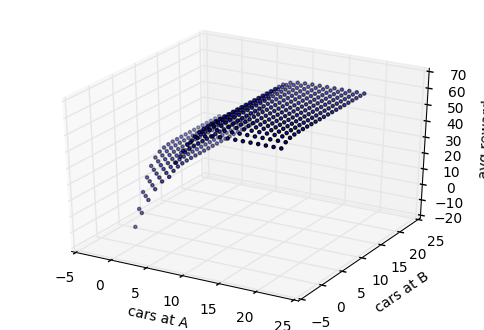Return¶

The return $G_t$ is defined as the total discounted reward:

$$G_t = R_{t+1} + \gamma R_{t+2} + \gamma^2 R_{t+3} + \dots = \sum_{k=0}^\infty \gamma^k R_{t+k+1}$$

Value functions¶

The state-value function $v_\pi (s)$ of an MDP is the expected return starting from state $s$, and then follow policy $\pi$

$$v_\pi (s) = \mathbb E_\pi[G_t \mid S_t=s] = \mathbb E \left[ \sum_{t'=t}^\infty \gamma^{t'-t} R_{t'+1} \mid S_t=s \right], s \in \mathcal S$$

The value function can be decomposed into an immediate part and the discounted value function of the successor states:

$$v_\pi(s) = \mathbb E[G_t \mid S_t =s] \\ = \mathbb E [R_{t+1}+γR_{t+2}+\gamma^2 R_{t+3} + \dots \mid S_t =s] \\ = \mathbb E [R_{t+1} + \gamma (R_{t+2}+\gamma R_{t+3} + \dots ) \mid S_t = s] \\ = \mathbb E [R_{t+1} + \gamma G_{t+1} \mid S_t = s] \\ = \mathbb E [ R_{t+1} \mid S_t = s]+ \gamma v_\pi(S_{t+1} )$$

so we have the Bellmann Equation (for deterministic Policies): $$v_\pi(s) = \mathcal R_s + \gamma \sum_{s'\in \mathcal S} P_{ss'}^\pi v_\pi (s')$$

Note: $v(s')$ are the values of the successor states of $s$, so in $\sum_{s'\in \mathcal S} P_{ss'} v(s')$ the weights $P_{ss'}$ of the values $v(s')$ are the transitions back in time.

Bellman Equation¶

also for non deterministic policies:

$$v_\pi(s) = \sum_a \pi(a \mid s) \left( \mathcal R_s^a + \gamma \sum_{s' \in \mathcal S} \mathcal P^a_{s,s'} v_\pi(s')\right)$$

Bellmann equations in vector-matrix form: $$\vec v = \mathcal R^{\pi} + \gamma P^{\pi} \vec v$$

Here we can direcly solve the Bellmann equation: $$\vec v = (I - \gamma \mathcal P)^{-1} \mathcal R$$

In :
def evaluate_policy(policy):
P_pi_transpose, reward = get_P_reward_for_policy(policy)

### Here we must use P_pi_transpose.T - transformation back in time to the previous state!
values = np.dot(np.linalg.inv(np.eye((MAX_CAPACITY+1)**2) - GAMMA * P_pi_transpose.T), reward)
return values
In :
policy_ = np.zeros(((MAX_CAPACITY+1)**2), dtype=int)
values = evaluate_policy(policy_)
plot3d_over_states(values, 'v')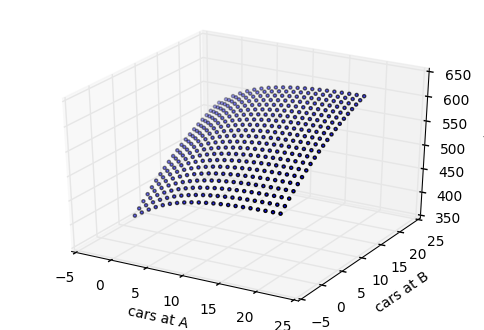Policy Evaluation¶

Computing the state-value function $v^\pi(s)$ for an arbitrary policy by an iterative application of Bellman expectation backup is called policy evaluation:

• $v_1 \rightarrow v_2 \rightarrow v_3 \rightarrow \dots \rightarrow v_\pi$
• using synchronous backups
• at each iteration $k+1$
• for all states $s \in \mathcal S$
• update $v_{k+1}(s)$ from $v_k(s')$, where $s'$ is a successor state of $s$
$$\vec v_{k+1} = \vec {\mathcal R}^\pi + \gamma {\mathcal P}^\pi \vec v_{k}$$

Optimal Value Function¶

$$v^* (s) = \max_\pi v_\pi(s)$$

The optimal value $v^*(s)$ of state $s \in \mathcal S$ gives the highest achievable expected return when the process is started from state $s$. The function $v^* : \mathcal S \rightarrow \mathbb R$ is called the optimal value function.

Policy Iteration¶

• Starting with an arbitrary policy
• Iteration until the values converge (to the optimal policy). Alternating sequence of
• Policy Evaluation : Compute the values of the policy
• Greedy Policy Improvement: Use the "best" action in each state.

For the greedy policy improvement we need the model (transition probabilities) if we are using the value function $v(s)$: For each action compute the probabilities of the next states and calculate the average value.

In :
def greedy_improve(values):
P_ = P.transpose(1, 0, 2) # we used the model for improvement
all_pure_states = np.eye((MAX_CAPACITY+1)**2)
new_states = np.dot(all_pure_states, P_)
average_new_values = np.dot(new_states.transpose(2,1,0), values)
average_new_values = average_new_values.T + Reward
policy_indices = np.argmax(average_new_values, axis=1)
policy = policy_indices - TRANSFER_MAX
return policy
In :
values_ = evaluate_policy_by_iteration(policy)
plot3d_over_states(values_, 'v')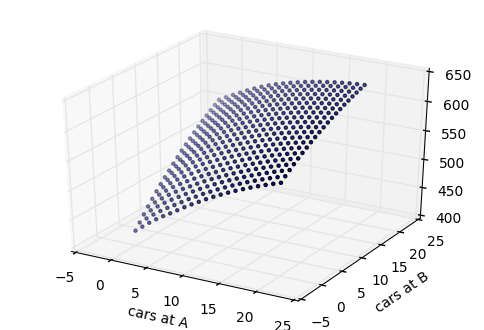In :
plot_policy(policy)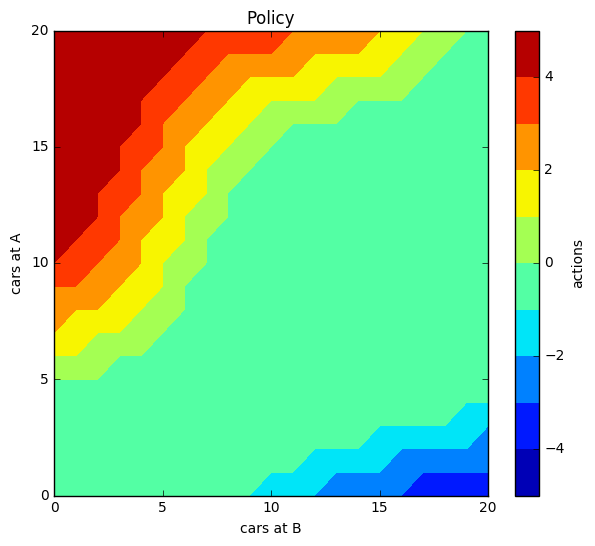Q-Function¶

The action-value function $q_\pi (s,a)$ of an MDP is the expected return starting from state $s$ with action $a$, and then follow policy $\pi$

$$q^\pi (s,a) = \mathbb E_\pi[G_t \mid S_t=s, A_t=a]$$

Optimal action-value function¶

$$q^* (s, a) = \max_\pi q^\pi(s, a)$$

The optimal action-value $q^*(s, a)$ of state-action pair $(s,a) \in \mathcal S \times \mathcal A$ gives the highest achievable expected return when the process is started from state $s$, and the first action chosen is $a$. The function $q^* : \mathcal S \times \mathcal A \rightarrow \mathbb R$ is called the optimal action-value function.

Connection between optimal value- and action-value function¶

$$v^*(s) = \max_{a \in \mathcal A} q^*(s,a), s \in \mathcal S$$$$q^*(s,a) = \mathcal R_s^a + \gamma\sum_{s' \in \mathcal S} P(s,a,s')v^*(s') ; s \in \mathcal S, a \in \mathcal A$$

Bellman Equation for Q¶

$$q_\pi(s, a) = \mathcal R_s^a + \gamma \sum_{s' \in \mathcal S} \mathcal P^a_{s,s'} \left( \sum_{a'\in \mathcal A} \pi(a' \mid s') q_\pi(s',a') \right)$$

for a deterministic policy: $$q_\pi(s, a) = \mathcal R_s^a + \gamma \sum_{s' \in \mathcal S} \mathcal P^a_{s,s'} q_\pi(s',\pi(s'))$$

$$\hat Q^\pi = \hat R + \gamma \mathcal P \vec Q^\pi_\pi$$

with

• $\vec \pi$: Policy vector
• Matricies $\hat Q^\pi, \hat R$ with entries $\cdot_{sa}$
• 3d Array $\mathcal P$ with entries $\cdot_{sas'}$
• $\vec Q^\pi_\pi = \vec v^\pi$

Policy Evaluation for Q¶

Computing the state-action-value function $q^\pi(s, a)$ for an arbitrary policy by an iterative application of Bellman expectation backup is also called policy evaluation:

• $q_1 \rightarrow q_2 \rightarrow q_3 \rightarrow \dots \rightarrow q_\pi$
• using synchronous backups
• at each iteration $k+1$
• for all states $s \in \mathcal S$ and $a \in \mathcal A$
• update $q_{k+1}(s, a)$ from $q_k(s', \cdot)$, where $s'$ is a successor state of $s$.
$$\hat Q^{k+1} = \hat R + \gamma \mathcal P \vec Q^k_{k}$$

Policy Iteration for Q¶

• Starting with an arbitrary policy
• Iteration until the values converge (to the optimal policy). Alternating sequence of
• Policy Evaluation : Compute the values of the policy
• Greedy Policy Improvement:
• $\pi'(s) = \text{arg} \max_{a \in \mathcal A} q^\pi(s,a)$
• Note: Here we don't use the (transition) model.
In :
def greedy_improve_Q(Q):
return np.argmax(Q, axis=1) - TRANSFER_MAX

policy = np.ones((MAX_CAPACITY+1)**2, dtype=int) * 0
Q = np.zeros([(MAX_CAPACITY+1)**2, 2*TRANSFER_MAX+1])

converged=False
while not converged:
new_Q = evaluate_policy_Q_by_iteration(policy, Q)
policy = greedy_improve_Q(Q)
if np.allclose(new_Q, Q):
converged = True
Q = new_Q

In :
plot_policy(policy)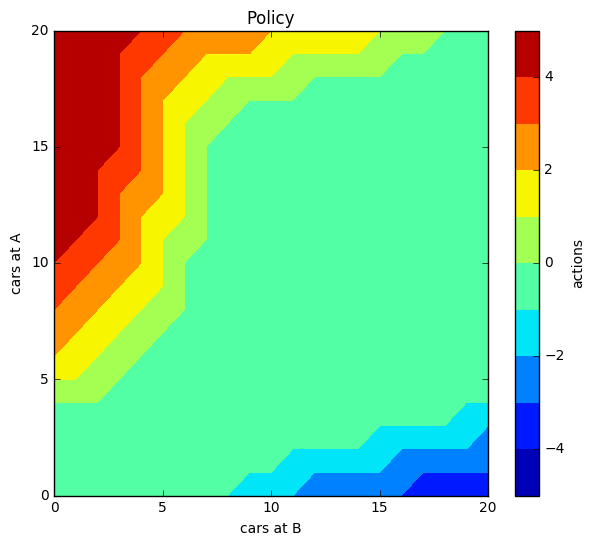Bellman Optimality Equation¶

$$v^*(s) = \max_a \mathbb E \left[ R_{t+1} + \gamma v^*(S_{t+1}) \mid S_t = s, A_t = a\right] \\ = \max_a \left(\mathcal R^a_{s} + \gamma \sum_{s'} P_{ss'}^a \mathcal v^*(S_{t+1}=s')\right)$$$$q^*(s, a) = \mathbb E \left[ R_{t+1} + \gamma \max_{a'} q^*(S_{t+1},A_{t+1}=a') \mid S_t = s, A_t = a\right] \\ = \mathcal R^a_{s} + \gamma \sum_{s'} P_{ss'}^a \mathcal \max_{a'} q^*(S_{t+1}=s',A_{t+1}=a')$$

Value Iteration¶

From the Bellmann optimality equation we can derive an iterative method to find the optimal value function:

$$v_{k+1}(S_t = s) = \max_a \mathbb E[R_{t+1} + \gamma v_k(S_{t+1}=s') \mid S_t=s, A_t=a] \\ = \max_a \left( \mathcal R_{s}^a + \gamma \sum_{s'} \mathcal P_{ss'}^a v_k(s')\right)$$
In :
converged = False
values = np.zeros((MAX_CAPACITY+1)**2)
P_ = P.transpose(1,2,0)
while not converged:
rs = Reward + GAMMA * np.dot(P_, values)
new_values = np.max(rs, axis = 1)
if np.allclose(values, new_values):
converged = True
values = new_values
In :
policy = np.argmax(Reward + GAMMA * np.dot(P_, values) , axis=1) - TRANSFER_MAX
plot_policy(policy)